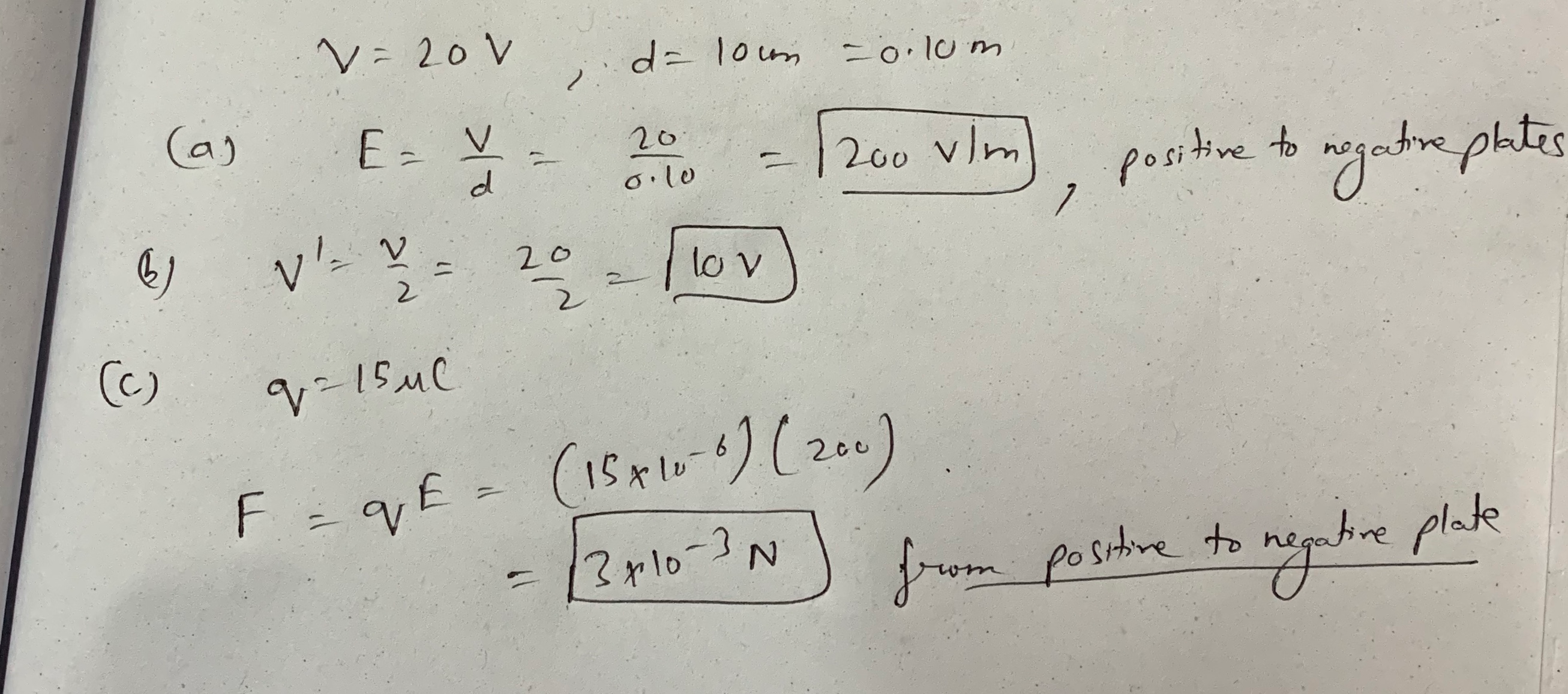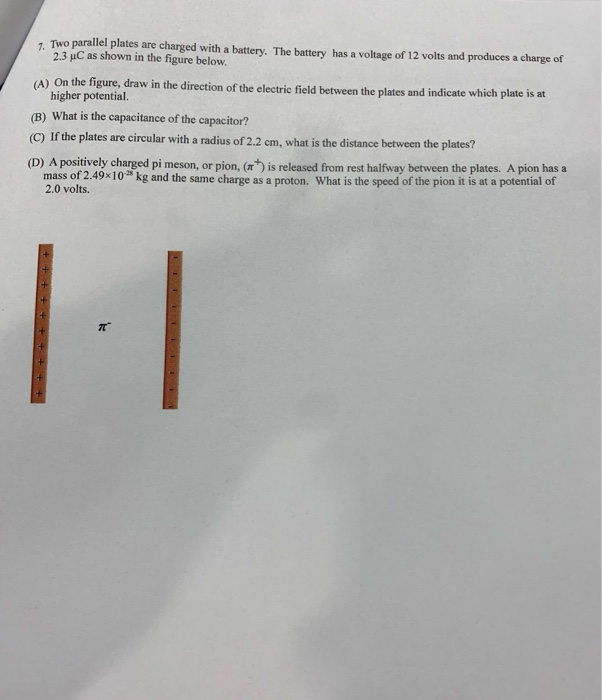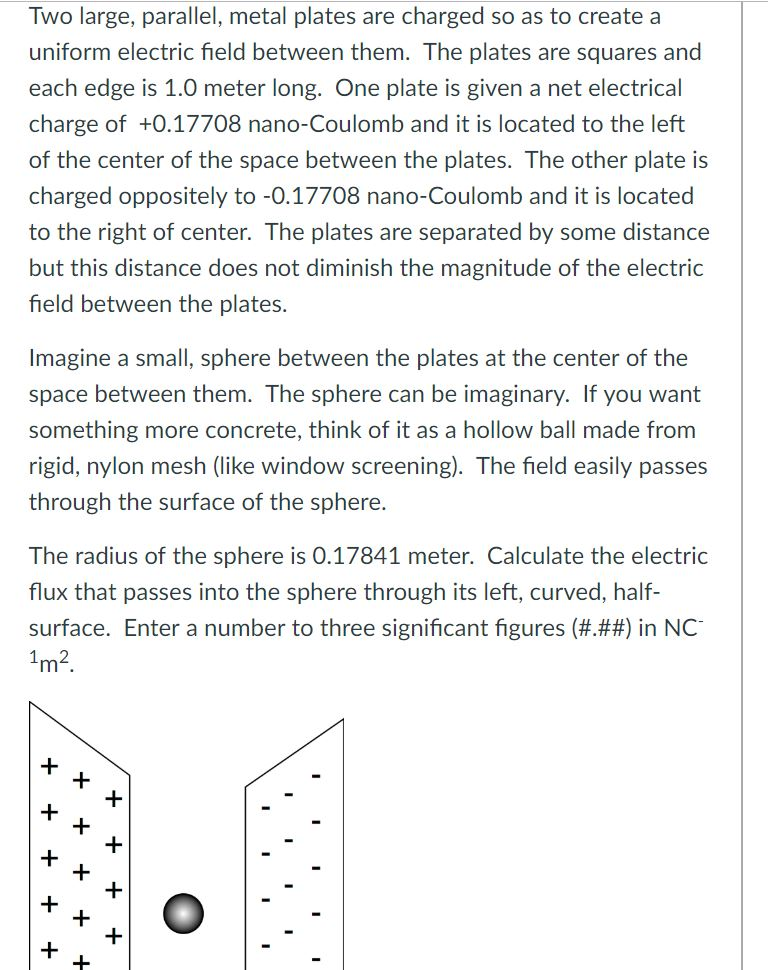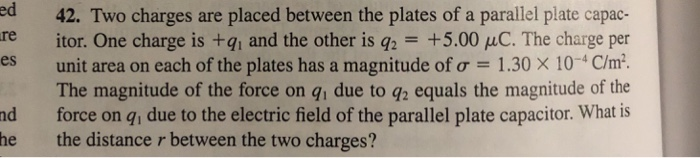Question

# The negative plate is grounded (0 volts) and the voltage between the plates is 20 volts....

The negative plate is grounded (0 volts) and the voltage between the plates is 20

volts. The distance between the plates is 10cm.

a.Compute the magnitude (volts per meter) and direction of the electric field

between the plates

b.What is the voltage halfway between the two plates

c.If a charge of 15μC is placed between the plates, compute the magnitude and

direction of the resultant Coulomb forceKindly upvote:)

#### Earn Coins

Coins can be redeemed for fabulous gifts.

Similar Homework Help Questions
• ### Two parallel plates are charged with a battery. The battery has a voltage of 12 volts...Two parallel plates are charged with a battery. The battery has a voltage of 12 volts and produces a charge of 23 uC as shown in the figure below. (A) On the figure, draw in the direction of the electric field between the plates and indicate which plate is at higher potential (B) What is the capacitance of the capacitor? (C) If the plates are circular with a radius of 2.2 cm. what is the distance between the plates? (D)...

• ### Two large, parallel, metal plates are charged so as to create a uniform electric field between...Two large, parallel, metal plates are charged so as to create a uniform electric field between them. The plates are squares and each edge is 1.0 meter long. One plate is given a net electrical charge of +0.17708 nano-Coulomb and it is located to the left of the center of the space between the plates. The other plate is charged oppositely to -0.17708 nano-Coulomb and it is located to the right of center. The plates are separated by some distance...

• ### ed 42. Two charges are placed between the plates of a parallel plate capac- itor. One...ed 42. Two charges are placed between the plates of a parallel plate capac- itor. One charge is +q1 and the other is q.-+5.00 μC. The charge per unit area on each of the plates has a magnitude or-1.3OX 10-4 C/m2 The magnitude of the force on qi due to q2 equals the magnitude of the force on q, due to the electric field of the parallel plate capacitor. What is re es nd he the distance r between the...

• ### Two charges are placed between the plates of a parallel plate capacitor. One charge is +q1...

Two charges are placed between the plates of a parallel plate capacitor. One charge is +q1 and the other is q2 = +4.58C. The charge per unit area on each plate has a magnitude of = 3.37 × 10-6 C/m2. The force on q1 due to q2 equals the force on q1 due to the electric field of the parallel plate capacitor. What is the distance r between the two charges?

• ### Two charges are placed between the plates of a parallel plate capacitor. One charge is +q1...

Two charges are placed between the plates of a parallel plate capacitor. One charge is +q1 and the other is q2 = +4.56C. The charge per unit area on each plate has a magnitude of = 8.63 × 10-3 C/m2. The force on q1 due to q2 equals the force on q1 due to the electric field of the parallel plate capacitor. What is the distance r between the two charges?

• ### Two parallel conducting plates are connected to a constant voltage source. The magntude of the electric...

Two parallel conducting plates are connected to a constant voltage source. The magntude of the electric field between the plates is 2506 N/C. If the voltage is doubled and the distance between the plates is reduced to 1 4 the original distance, what is the magnitude of the new electric field? Answer in units of N/C. A pair of oppositely charged parallel plates is separated by 5.36 mm. A potential difference of 582 V exists between the plates. What is...

• ### A free electron is placed between two large, parallel, nonconducting plates that are horizontal and 7.83...

A free electron is placed between two large, parallel, nonconducting plates that are horizontal and 7.83 cm apart. One plate has a uniform positive charge; the other has an equal amount of uniform negative charge. The force on the electron due to the electric field Upper E Overscript right-arrow EndScripts between the plates balances the gravitational force on the electron. What are (a) the magnitude of the surface charge density on the plates and (b) the direction of Upper E...

• ### A parallel-plate capacitor has capacitance C0 = 7.00 pF when there is air between the plates. The separation between the plates is 1.10 mm.

A parallel-plate capacitor has capacitance C0 = 7.00 pF when there is air between the plates. The separation between the plates is 1.10 mm.  a. What is the maximum magnitude of charge Q that can be placed on each plate if the electric field in the region between the plates is not to exceed 3.00 x 104 V/m? b. A dielectric with K = 2.60 is inserted between the plates of the capacitor, completely filling the volume between the plates. Now...

• ### Two parallel plates, each of area 3.37 cm2, are separated by 4.80 mm. The space between...

Two parallel plates, each of area 3.37 cm2, are separated by 4.80 mm. The space between the plates is filled with air. A voltage of 6.25 V is applied between the plates. Calculate the magnitude of the electric field between the plates. Tries 0/20 Calculate the amount of the electric charge stored on each plate. Tries 0/20 Now distilled water is placed between the plates and the capacitor is charged up again to the same voltage as before. Calculate the...

• ### A parallel-plate capacitor has capacitanceC0 = 7.80 pF when there is air between the plates. The...

A parallel-plate capacitor has capacitanceC0 = 7.80 pF when there is air between the plates. The separation between the plates is 1.10 mm . What is the maximum magnitude of charge that can be placed on each plate if the electric field in the region between the plates is not to exceed 3.00×104 V/m ? A dielectric with K = 2.80 is inserted between the plates of the capacitor, completely filling the volume between the plates. Now what is the...